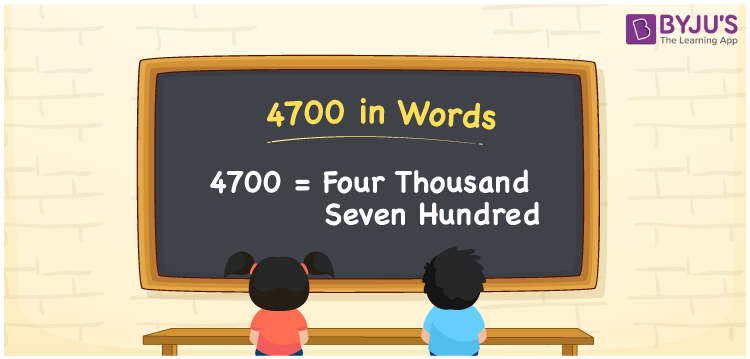# 4700 in Words

4700 in words can be written as Four Thousand Seven Hundred. Here students will learn briefly regarding numbers and how to count it. For example, if you buy 3 shirts for Rs. 4700, then you can say that “I bought 3 shirts for Four Thousand Seven Hundred Rupees”. Get to know more about how to write numbers in words using the English alphabet. Hence, 4700 can be read as “Four Thousand Seven Hundred” in English.

 4700 in words Four Thousand Seven Hundred Four Thousand Seven Hundred in Numbers 4700

## 4700 in English Words## How to Write 4700 in Words?

4700 in the form of a place value chart is shown below. There are four digits in 4700 whose place value is clearly mentioned in the table given here.

 Thousands Hundreds Tens Ones 4 7 0 0

The expanded form of 4700 is:

4 x Thousands + 7 × Hundred + 0 × Ten + 0 × One

= 4 x 1000 + 7 × 100 + 0 × 10 + 0 × 1

= 4000 + 700

= 4700

= Four Thousand Seven Hundred

Therefore, 4700 in words is written as Four Thousand Seven Hundred.

4700 is a natural number that precedes 4701 and succeeds 4699.

4700 in words – Four Thousand Seven Hundred

Is 4700 an odd number? – No

Is 4700 an even number? – Yes

Is 4700 a perfect square number? – No

Is 4700 a perfect cube number? – No

Is 4700 a prime number? – No

Is 4700 a composite number? – Yes

## Frequently Asked Questions on 4700 in Words

Q1

### How can 4700 be written in words?

4700 can be written in words as “Four Thousand Seven Hundred”.
Q2

### Write Four Thousand Seven Hundred in numbers.

Four Thousand Seven Hundred can be written in numbers as 4700.
Q3

### Is 4700 an even number?

Yes, 4700 is an even number as it is completely divisible by 2.